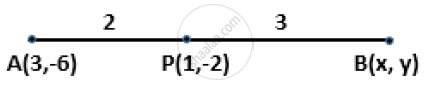Share

# P(1, -2) is a Point on the Line Segment A(3, -6) and B(X, Y) Such that Ap : Pb is Equal to 2 : 3. Find the Coordinates of B. - Mathematics

Course

#### Question

P(1, -2) is a point on the line segment A(3, -6) and B(x, y) such that AP : PB is equal to 2 : 3. Find the coordinates of B.

#### SolutionGiven, P(1, -2), A(3, -6) and B(x,y)

AP:PB = 2:3

Hence, coordinates of P = ((2xxx+3xx3)/(2+3), (2xxy+3xx(-6))/(2+3)) = ((2x + 9)/5, (2y- 18)/5)

But, the coordinates of P are (1, 2).

∴ (2x + 9)/5 = 1 and (2y - 18)/5 = -2

⇒ 2x + 9 = 5 and 2y - 18 =-10

⇒ 2x = -4 and 2y = 8

⇒ x = -2 and y = 4

Hence, the coordinates of B are (-2,4).

Is there an error in this question or solution?